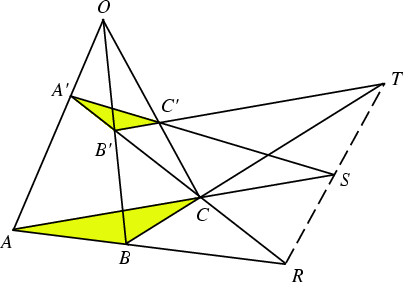## Desargues' TheoremIf the three straight Lines joining the corresponding Vertices of two Trianglesandall meet in a point (the Perspective Center), then the three intersections of pairs of corresponding sides lie on a straight Line (the Perspective Axis). Equivalently, if two Triangles are Perspective from a Point, they are Perspective from a Line.

Desargues' theorem is dual to Pappus's Hexagon Theorem according to the Duality Principle of Projective Geometry.

See also Duality Principle, Pappus's Hexagon Theorem, Pascal Line, Pascal's Theorem, Perspective Axis, Perspective Center, Perspective Triangles

References

Coxeter, H. S. M. and Greitzer, S. L. Geometry Revisited. Washington, DC: Math. Assoc. Amer., pp. 70-72, 1967.

Ogilvy, C. S. Excursions in Geometry. New York: Dover, pp. 89-92, 1990.